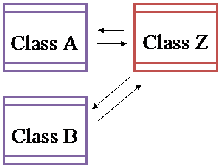## Tuesday, October 27, 2009

### Asynchronous Threading in Blackberry

Assume that you are having a class called Class A it needs to call Class Z as a thread. Therefore you can  implement class Z from Runnable interface and implement your code in run().

Ex:-
`public class ClassZ implements Runnable {`
` `
`    public static final int METHOD1 =1;`
`    public static final int METHOD2= 2;    `
` `
`    int code;`
`    `
`    public ClassZ(int code)`
`    { this.code=code; }     `
` `
`    private void method1()`
`    { //Code for Method1 }`
` `
`    private void method2()`
`    { //Code for Method2 }`
` `
`    public void run() {`
`    `
`        switch (code)`
`        {`
`        case ClassZ.METHOD1 :`
`            method1();`
`            break;`
`        case ClassZ.METHOD2:`
`            method2();`
`            break;`
`        }`
`    }`
` `
`    public void runThread() {`
`    thread = new Thread(this);`
`    thread.start();`
`    }`
`}`

If you start the Thread using ClassZ it runs ClassZ’s run method. Therefore  if you have several method in side the class you need to have a variable telling which method you want to run. Thus in here i used code variable to  keep the information about the method which i want to run. And also we wrote a method to start the thread called runThread().

`public Class ClassA `
`{`
`    method()`
`    {`
`        // Initialize the ClassZ by passing which`
`        // method we used to invoke as thread`
`        ClassZ clsz = new ClassZ(ClassZ.method1);`
`        clsz.runThread();`
`    }`
`}`

Sometimes we want to do something after finish executing the thread. But in here there are no return vales in a thread. therefore we use mechanism called callBack().Assume that you are calling the thread class  from ClassA or ClassB and want to get some feedback according the caller. Therefore we can change the code as below.

`public class ClassZ implements Runnable {`
` `
`    public static final int METHOD1 =1;`
`    public static final int METHOD2= 2;    `
` `
`    int code;`
`    Object object;`
`    `
`    public ClassZ(int code,Object object)`
`    { this.code=code;`
`      this.object=object;  `
`    }     `
` `
`    private void method1()`
`    { //Code for Method1`
`        callBack();`
`    }`
` `
`    private void method2()`
`    { //Code for Method2 `
`        callBack();`
`    }`
` `
`    public void run() {`
`    `
`        switch (code)`
`        {`
`        case ClassZ.METHOD1 :`
`            method1();`
`            break;`
`        case ClassZ.METHOD2:`
`            method2();`
`            break;`
`        }`
`    }`
` `
`    public void runThread() {`
`    thread = new Thread(this);`
`    thread.start();`
`    }`
` `
`    void callBack()`
`    {`
`        if (object instanceof ClassA) {`
`            ((ClassA) object).callBack();`
`        }`
`        `
`        else if (object instanceof ClassB) {`
`            ((ClassB) object).callBack();`
`        }`
`    }`
`}`
If method finished executed it invoke callBack(). The method check the object instance and invoke relevant callBack() in the class.

`public Class ClassA `
`{`
`    method()`
`    {`
`        // Initialize the ClassZ by passing which`
`        // method we used to invoke as thread and `
`        // object itself`
`        ClassZ clsz = new ClassZ(ClassZ.method1,this);`
`        clsz.runThread();`
`    }`
` `
`    public void callBack()`
`    {`
`        // code after executing the`
`        // thread`
`    }`
`}`

#### 1 comment:Anonymous said...

good example.